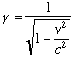## The Gamma Factor

At this point, you may be wondering why you've never noticed the effects of length contraction and time dilation in everyday life. For example, according to what I just got done saying, if you drive from Omaha to Kansas City and back, you should have to reset your watch when you get home. After all, while you're driving your watch should run slower than all the clocks which are sitting still in your home. If your watch says 3:00 when you get back, then all your clocks at home should read a later time. Why haven't you noticed this before?

The answer is that the effect depends on how fast you're going, and you're going very slow. (You and that Missouri State Trooper may think you're driving fast, but as far as the theory of relativity is concerned, you're driving very slow.) The effects of time dilation and length contraction are only noticeable if you're traveling close to the speed of light, which is about 186,300 miles per second (or 300,000,000 meters/second).

Mathematically, relativistic effects are described by a factor which physicists normally denote by the Greek letter gamma. This factor depends on the speed at which an object is traveling. For example, if a meter stick (proper length 1 meter) is moving past us very rapidly, its length in our reference frame is 1 meter divided by gamma. If a clock traveling from point A to point B measures 3 seconds during its trip, then in our reference frame, the trip takes 3 seconds times gamma.

To see why we never notice relativistic effects in real life, let's look at the formula for gamma:The key here is the v2/c2 in the denominator. v is the velocity of the object we're talking about, while c is the speed of light. Since the velocity of any normal-sized object is much less than the speed of light, v/c is very small, and when we square this it's smaller still. So, gamma is, for all practical purposes, 1 for any normal sized object you've ever seen. And since we calculate relativistic effects by multiplying or dividing by gamma, at normal speeds lengths and times are virtually unchanged. To illustrate this, here's a table of the value of gamma for different speeds. The last column is the length of a meter stick moving at this speed, in meters. (This is just 1 meter, divided by gamma.)

 Speed Speed (miles/hour) Gamma Length 0 0 1 1 20 meters/second 45 1.0000000000000022 .9999999999999978 100,000 m/s 224,000 1.000000056 .999999944 .1 c (30 million m/s) 67 million 1.005 .995 .9 c 600 million 2.29 .44 .999 c 670 million 22.4 .045 c 670 million Infinite 0

In the first column, c is the speed of light again, so that .9 c means nine tenths of the speed of light, for example. For reference, a Saturn V rocket used to take astronauts to the moon traveled at a speed of around 25,000 miles per hour. So you can see for an object at any reasonable speed, gamma is indistinguishable from 1, so that lengths and times are virtually undistorted. For all practical purposes, relativistic effects such as length contraction and time dilation only occur in science fiction novels (in which spaceships are much faster than a Saturn V) and in particle physics, where electrons and protons are routinely accelerated to speeds very close to the speed of light. The effects don't show up flying from Chicago to Denver.